# Test: Buoyancy & Floatation - 1

## 10 Questions MCQ Test GATE Civil Engineering (CE) 2023 Mock Test Series | Test: Buoyancy & Floatation - 1

Description
Attempt Test: Buoyancy & Floatation - 1 | 10 questions in 30 minutes | Mock test for Mechanical Engineering preparation | Free important questions MCQ to study GATE Civil Engineering (CE) 2023 Mock Test Series for Mechanical Engineering Exam | Download free PDF with solutions
QUESTION: 1

### A solid sphere of radius R and average mass density ρi is completely submerged in a tank filled with a liquid of mass density ρw with ρi > ρw- The sphere is held in place by string attached to the bottom of tank. The tension in the string is

Solution:

Weight of sphere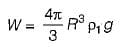Buoyant force,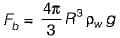since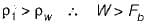The tension in the string, T, compensates the difference between the weight and the buoyant force and hence W = T + Fb

so,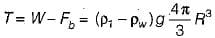QUESTION: 2

### If B = Center of buoyancy, G = Center of gravity and M= Metacenter, of afloating body, the body will be in stable equilibrium if

Solution:

For a floating body
Metacentre above centre of gravity→ Stable Equilibrium
Metacentre coincides centre of gravity→ Neutral Equilibrium
Metacentre below centre of gravity→ Unstable Equilibrium
For a submerged body
Centre of buoyancy above centre of gravity→ Stable Equilibrium
Centre of buoyancy coincides centre of gravity→ Neutral Equilibrium
Centre of buoyancy below centre of gravity→ Unstable Equilibrium

QUESTION: 3

### A metal block is thrown into deep lake. As it sinks deeper in water, the buoyant force acting on it

Solution:

Buoyant force equals the weight of water which has been displaced by an object.
Since, irrespective of the metal block position, the volume displaced by metal is same, hence, buoyant force remains the same.

QUESTION: 4

When a block of ice floating on water in a container melts, the level of water in the container

Solution: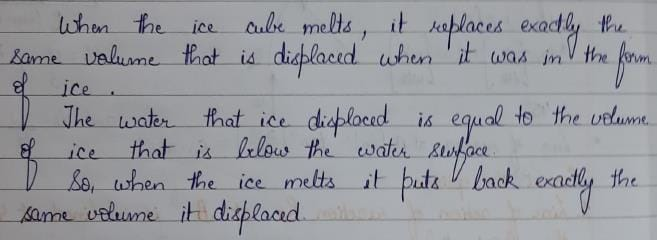QUESTION: 5

When a ship enters sea from a river one can expect it

Solution:
QUESTION: 6

A large metacentric height in a vessel

Solution:
QUESTION: 7

For warship, metacentric height of a ship should vary between

Solution:
QUESTION: 8

The metacentric height of a passenger ship is kept water lower than that of a naval or a cargo ship. This is because

Solution: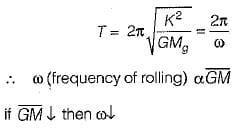QUESTION: 9

The buoyant force is

Solution:

A body submerged in a fluid experiences an upward thrust due to fluid pressure. This force is called buoyant force and a body immersed in a fluid is buoyed or lifted up a force equal to the weight of the fluid displaced by the body. The body apparently loses as much of its weight as the weight of the fluid displaced by it.

QUESTION: 10

The metacenter is

Solution:

Metacenter is the point of inter section of normal axis of the body with the new line of action of buoyant force when body is tilted.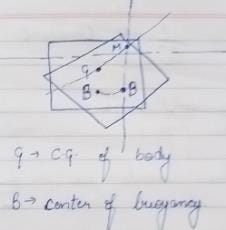Use Code STAYHOME200 and get INR 200 additional OFF Use Coupon Code